Stack of clipart images

A stack, or pile, of clipart images. This image was designed by James Seaman for use as a default image…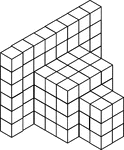108 Stacked Congruent Cubes

Illustration of 108 congruent cubes stacked at various heights. A 3-dimensional representation on a…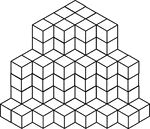117 Stacked Congruent Cubes

Illustration of 117 congruent cubes stacked in columns of one, four, and six. A 3-dimensional representation…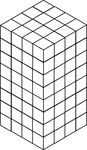128 Stacked Congruent Cubes

Illustration of 128 congruent cubes stacked so they form a rectangular solid that measures 4 by 4 by…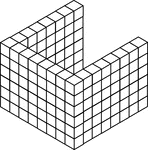132 Stacked Congruent Cubes

Illustration of 132 congruent cubes stacked in 22 columns of 6 in the shape of a U. A 3-dimensional…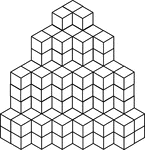154 Stacked Congruent Cubes

Illustration of 154 congruent cubes stacked in columns increasing from one to four. A 3-dimensional…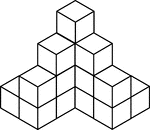16 Stacked Congruent Cubes

Illustration of 16 congruent cubes stacked at various heights. A 3-dimensional representation on a 2-dimensional…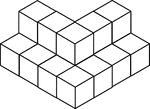17 Stacked Congruent Cubes

Illustration of 17 congruent cubes stacked in ones and twos in the shape of a V. A 3-dimensional representation…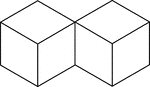2 Congruent Cubes

Illustration of two congruent cubes that are tangent along an edge. A 3-dimensional representation on…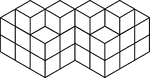20 Stacked Congruent Cubes

Illustration of 20 congruent cubes stacked in twos and threes. A 3-dimensional representation on a 2-dimensional…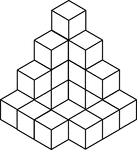20 Stacked Congruent Cubes

Illustration of 20 congruent cubes stacked at various heights. A 3-dimensional representation on a 2-dimensional…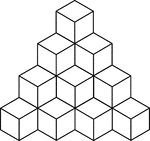20 Stacked Congruent Cubes

Illustration of 20 congruent cubes stacked at heights increasing from 1 to 4 cubes. A 3-dimensional…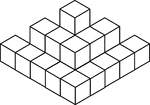22 Stacked Congruent Cubes

Illustration of 22 congruent cubes stacked in ones, twos, and threes. A 3-dimensional representation…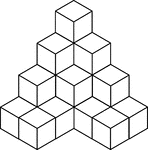22 Stacked Congruent Cubes

Illustration of 22 congruent cubes stacked at various heights. A 3-dimensional representation on a 2-dimensional…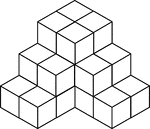24 Stacked Congruent Cubes

Illustration of 24 congruent cubes stacked at various heights to resemble steps. A 3-dimensional representation…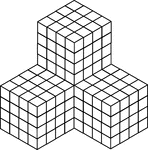256 Stacked Congruent Cubes

Illustration of 256 congruent cubes stacked so they form 4 larger cubes that measures 4 by 4 by 4 each.…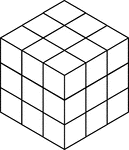27 Stacked Congruent Cubes

Illustration of 27 congruent cubes stacked to resemble a larger cube that measures three by three by…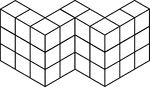27 Stacked Congruent Cubes

Illustration of 27 congruent cubes stacked at various heights in the shape of a W. A 3-dimensional representation…28 Congruent Cubes Placed in the Shape of a Square

Illustration of 28 congruent cubes placed in the shape of a square. A 3-dimensional representation on…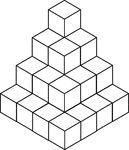30 Stacked Congruent Cubes

Illustration of 30 congruent cubes stacked in decreasing heights. A 3-dimensional representation on…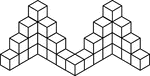33 Stacked Congruent Cubes

Illustration of 33 congruent cubes stacked at various heights in a zigzag pattern. A 3-dimensional representation…35 Stacked Congruent Cubes

Illustration of 35 congruent cubes stacked in ones and twos in the shape of a W. A 3-dimensional representation…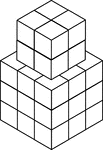35 Stacked Congruent Cubes

Illustration of 35 congruent cubes stacked at various heights. A 3-dimensional representation on a 2-dimensional…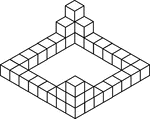36 Stacked Congruent Cubes

Illustration of 36 congruent cubes stacked at various heights with outer edges forming a square. A 3-dimensional…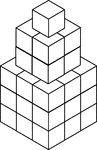36 Stacked Congruent Cubes

Illustration of 36 congruent cubes stacked to resemble a 1 by 1 by 1 cube on a 2 by 2 by 2 cube on a…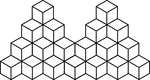39 Stacked Congruent Cubes

Illustration of 39 congruent cubes stacked at various heights. A 3-dimensional representation on a 2-dimensional…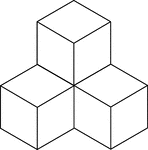4 Stacked Congruent Cubes

Illustration of 4 congruent cubes stacked in ones and twos. A 3-dimensional representation on a 2-dimensional…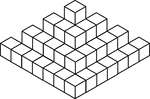50 Stacked Congruent Cubes

Illustration of 50 congruent cubes stacked at various heights. A 3-dimensional representation on a 2-dimensional…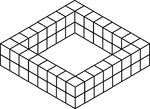56 Stacked Congruent Cubes

Illustration of 56 congruent cubes stacked in twos in the shape of a square. A 3-dimensional representation…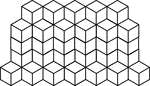56 Stacked Congruent Cubes

Illustration of 56 congruent cubes stacked in heights of 1, 4, and 5 cubes that form a zigzag pattern.…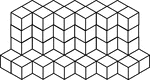57 Stacked Congruent Cubes

Illustration of 57 congruent cubes stacked in heights of 1 and 5 cubes that form a zigzag pattern. A…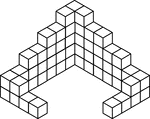59 Stacked Congruent Cubes

Illustration of 59 congruent cubes stacked at various heights. A 3-dimensional representation on a 2-dimensional…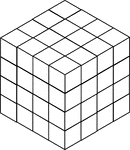64 Stacked Congruent Cubes

Illustration of 64 congruent cubes stacked so they form a cube that measures 4 by 4 by 4. A 3-dimensional…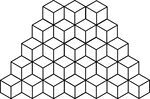65 Stacked Congruent Cubes

Illustration of 65 congruent cubes stacked at heights increasing from 1 to 5 cubes. A 3-dimensional…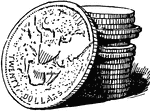Double Eagles Stack

A stack of double eagle coins. A Double Eagle is a gold coin of the United States with a denomination…